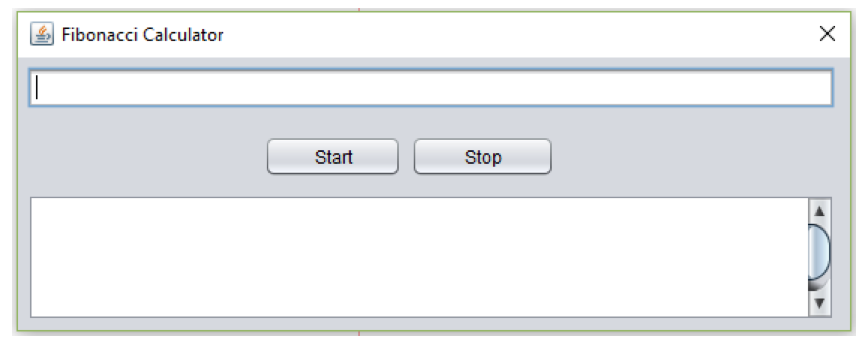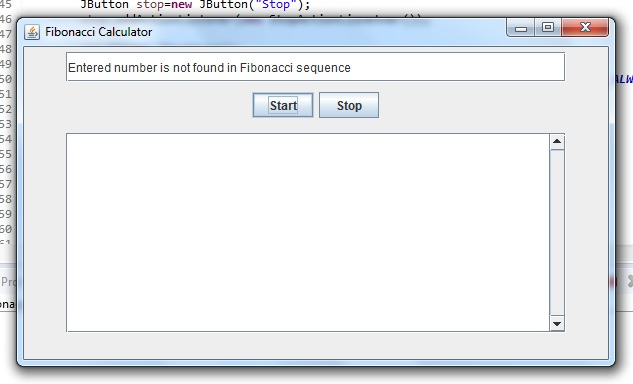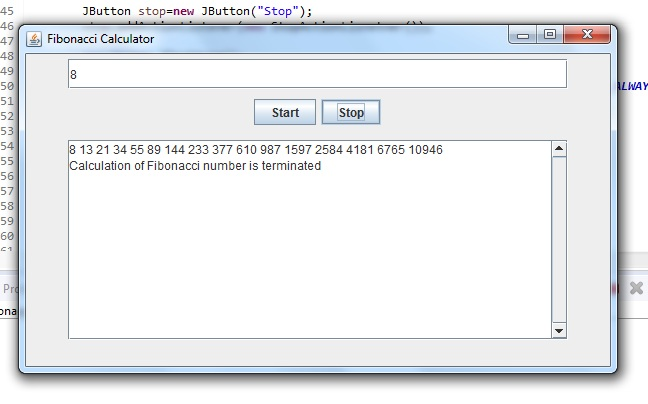# Answered! Implement a Java GUI multi-threaded application that computes a Fibonacci number. The expected screen shot…

Implement a Java GUI multi-threaded application that computes a Fibonacci number. The expected screen shot of the application is shown belowDon't use plagiarized sources. Get Your Custom Essay on
Answered! Implement a Java GUI multi-threaded application that computes a Fibonacci number. The expected screen shot…
GET AN ESSAY WRITTEN FOR YOU FROM AS LOW AS \$13/PAGE

Application User Interface:

A JTextField control – It accepts an integer number from the user for computing the Fibonacci value from this number.

A JButton control labelled as “Start” – When the user presses this button, the application will make sure that a valid number is entered by the user before computing the Fibonacci value from this number. If the number entered is not specified or invalid (i.e., not an integer value), the user will be warned with a message dialog. Once the number entered is validated, the Fibonacci calculation using this number will begin.   Refer to the section “Calculating Fibonacci Number” for further requirements.

A JButton control labelled as “Stop” – When the user presses this button, the calculation of Fibonacci number will be stopped. The message, “Calculation of Fibonacci number is terminated”, will be displayed in the JTextArea control as described below.

A JTextArea control is used to display any messages as well as the Fibonacci calculation progress. The JTextArea control must be scrollable both horizontally and vertically.

Calculating Fibonacci Number:

Create a worker thread to compute the Fibonacci number.

The application must use recursion to calculate the Fibonacci number.

For each recursion call during the Fibonacci calculation, the application must display the current calculated value in the JTextArea control as shown above. In order for the messages to appear and the user will be able to see them, make sure to add a call to sleep for 1 second.

A mechanism to terminate this thread when the “Stop” button is pressed must be synchronized (i.e., protected for concurrent accesses)

The application must be able to handle large number and exceptions generated due to data overflow. The error messages must be presented in the JTextArea control before the calculation of the Fibonacci number is terminated.

Following is the combined code for the above requested functionalities. Comments are added at required places of the code.

New Update: All the above requested functionalities have been included and is found to be functional upto this point.

– There was some code changes to the last code, use this one as the finished one.

UPDATED WITH ALL FUNCTIONALITIES

import java.awt.Dimension;
import java.awt.event.ActionEvent;
import java.awt.event.ActionListener;
import java.awt.event.FocusEvent;
import java.awt.event.FocusListener;

import javax.swing.JButton;
import javax.swing.JFrame;
import javax.swing.JPanel;
import javax.swing.JScrollPane;
import javax.swing.JTextArea;
import javax.swing.JTextField;
import javax.swing.ScrollPaneConstants;

public class Fibonacci extends JFrame{
StringBuilder str;
JTextField input;
JTextArea result;
JScrollPane hscroll;
JScrollPane vscroll;
boolean condition;
JScrollPane scrollPane;
long nextStart;
public Fibonacci(){

/*Below are the GUI items that are added on the JFrame.
* They are initialized with the required parameters based on the requirements
*/

JPanel panel1=new JPanel();
input=new JTextField();
input.setPreferredSize(new Dimension(500,30));

JButton start=new JButton(“Start”);

JButton stop=new JButton(“Stop”);

result=new JTextArea();
//result.setPreferredSize(new Dimension(500,200));
scrollPane = new JScrollPane(result,ScrollPaneConstants.VERTICAL_SCROLLBAR_ALWAYS,
ScrollPaneConstants.HORIZONTAL_SCROLLBAR_AS_NEEDED);

scrollPane.setPreferredSize(new Dimension(500,200));
JPanel panel2=new JPanel();
JPanel panel3=new JPanel();
}

public static void main(String[] args) {
JFrame frame = new Fibonacci();
frame.setTitle(“Fibonacci Calculator”);
frame.setSize(600,350);
frame.setLocationRelativeTo(null); // Center the frame
frame.setDefaultCloseOperation(JFrame.EXIT_ON_CLOSE);
frame.setVisible(true);
}
// The below class deals with making the textmsg in textbox hidded or visible
// based on whether its in focus or not.

public class TextClearActionListener implements FocusListener {

@Override
public void focusGained(FocusEvent arg0) {
input.setText(null);

}

@Override
public void focusLost(FocusEvent arg0) {

}

}

// Implementing the below ActionListner for Starting the sequence

public class StartActionLisnetner implements ActionListener {
boolean ck;
@Override
public void actionPerformed(ActionEvent arg0) {
try{
Long inx=Long.parseLong(input.getText());
ck=checkFibonacci((inx));
if(ck){
condition=true;
t.start();
}
else{
}
}catch(Exception e){
result.append(“n”+e.toString());
}

}

}
public boolean checkFibonacci(long input){
long i=0;
long x=0;
long y=1;

if(input !=0 || input!=1){
i=fib(x,y);
while(i<=input){

if(input==i){
nextStart=fib(y,i);
return true;
}
x=y;
y=i;
i=fib(x,y);
}
}
if(input==0){
nextStart=1;
return true;
}
return false;

}
public long fib(long x,long y){
return x+y;
}

// Implementing the below ActionListner for Stopping the sequence

public class StopActionLisnetner implements ActionListener {

@Override
public void actionPerformed(ActionEvent e) {
condition=false;
result.append(“nCalculation of Fibonacci number is terminated”);
//The above message getting displayed when the Stop button is pressed
}

}

public class CalculateFibonacci implements Runnable {
long x;
long y;

CalculateFibonacci(long x,long y){
this.x=x;
this.y=y;
}
@Override
public void run() { //is called when Thread start() is called

try{
result.append(Long.toString(x)); //Adding the initial 0 to series
result.append(Long.toString(y)); //Adding the initial 1 to series
calculate(x,y);

}catch(Exception e){
//In case of any exception the message is going to be shown in textArea

result.append(“n”+e.toString());
}

}

//This method is called recursively. This is the method that is calculating the
// the next value in the fibonacci sequence
public void calculate(long x,long y){
try {
} catch (InterruptedException e) {
// TODO Auto-generated catch block
//e.printStackTrace();
result.append(“n”+e.toString()); // The exception message is added in the textarea
}
while(condition){ //Checking to see that if Stop button is not pressed
result.append(” “);
result.append(Long.toString(x+y));
calculate(y,y+x);
}

}
}

}

Below are the screenshots from the dry run of the above code.